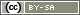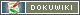# Placing Elements Side by Side

This chapter demonstrates how to place elements in the questionnaire side by side. For example, an image nest to a question or two questions side by side.

Important: If you'd like to place two questions side-by-side that contain the same items (for an Fishbein scale, for example), please read Combined Questions.

Example 1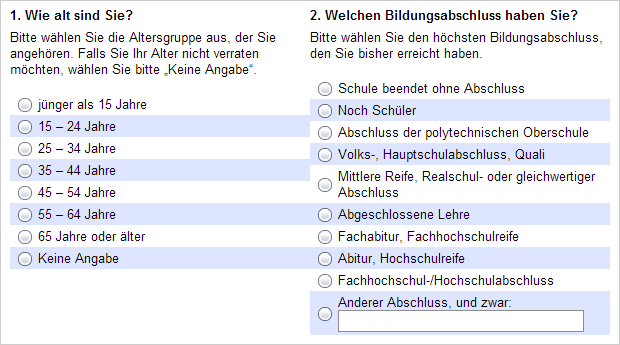Example 2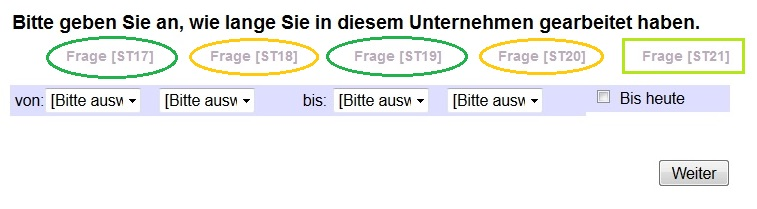For a step-by-step manual for the above example, please read Place Dropdowns Side by Side.

## Basics: Tables

The basis for a two-or-more-column layout is a HTML table. A simple HTML-table is shown below. Underneath the HTML-code for a table with 3 columns of the same width and two rows is displayed.

 Column 1 Column 2 Column 3 Row 1 Cell 1/1 Cell 1/2 Cell 1/3 Row 2 Cell 2/1 Cell 2/2 Cell 2/3
```<table>      <!-- This is where the table begins -->
<colgroup>   <!-- This is to define the widths of the columns -->
<col width="33%">   <!-- Column 1 has a width of 33%, col = column -->
<col width="33%">
<col width="34%">
</colgroup>
<tr>         <!-- This is the beginning of row 1, tr = table row -->
<td>cell 1/1</td>  <!-- This is cell 1/1, td = table data -->
<td>cell 1/2</td>  <!-- All rows now have 3 cells -->
<td>cell 1/3</td>
</tr>
<tr>
<td>cell 2/1</td>
<td>cell 2/2</td>
<td>cell 2/3</td>
</tr>
</table>```

Hint: The specification `<colgroup>` is optional. Although it usually is useful to define the column widths exactly. Instead of percentages you may also enter the width in pixels (e.g. `width=“150px”`).

Hint: Instead of using tables, you can use CSS for layout purposes. However, this requires advanced HTML skills.

## Using tables in the questionnaire

This is the theory how to use tables to place two questions side by side:

 Column 1 Column 2 Row 1 Question 1 Question 2

Now, how do we get the questions into the table? This is done easily by writing the table “around” the questions. To do so, you interrupt the table code at the point where the question should be. Once again, below the HTML-code for a table with one row and two columns.

Hint: The `table`-tag in the following example carries some more attributes. Those are necessary to a) prevent the browser from drawing a line around the table cells (`border=“0”`) and b) use the whole page for the table (`width=“100%”`).

```<table width="100%" cellspacing="0" cellpadding="0" border="0">
<colgroup>
<col width="50%">
<col width="50%">
</colgroup>
<tr>
<td>Question 1 goes here</td>
<td>Question 2 goes here</td>
</tr>
</table>```

By interrupting the HTML code in the table cells (`td`), you get three parts:

Part 1

```<table width="100%" cellspacing="0" cellpadding="0" border="0">
<colgroup>
<col width="50%">
<col width="50%">
</colgroup>
<tr>
<td> //Question 1 goes here```

Part 2

```    </td>
<td>//Question 2 goes here```

Part 3

```    </td>
</tr>
</table>```

Between those parts you can position any questions or other things like images or texts on the compose questionnaire page. (To do so, drag and drop the “HTML code”-brick from the right onto your questionnaire page, put the table parts into an “HTML code”-field for each and add your questions in between)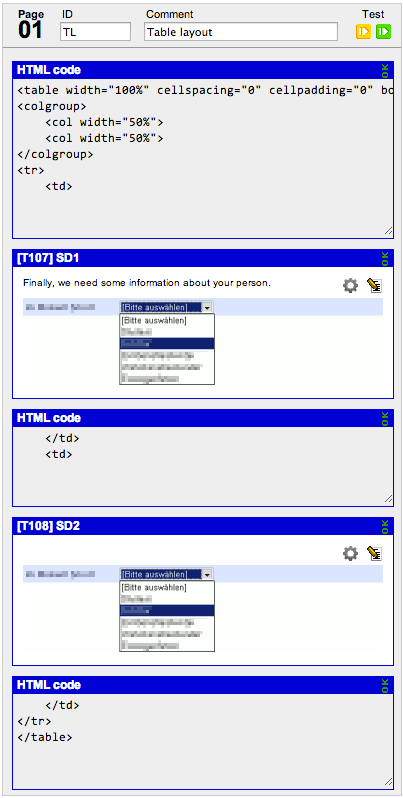This is how the example would look like: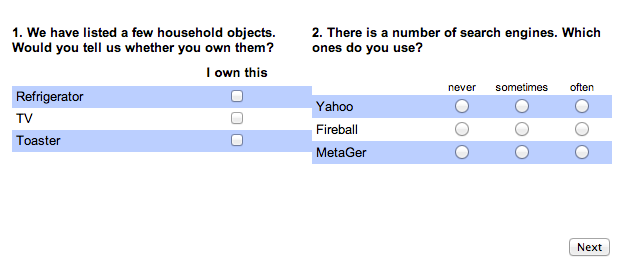## Optimize the display

As you see in example one, the output may need to be optimized.

### Spacing

If you need spacing between the individual questions, add an empty cell in between the questions (in part two).

```<table width="100%" cellspacing="0" cellpadding="0" border="0">
<colgroup>
<col width="45%">
<col width="10%"> <!-- A third column was added, note its smaller width as it will not contain any content -->
<col width="45%">
</colgroup>
<tr>
<td>Question 1</td>
<td></td>         <!-- This is an empty cell used as a spacer -->
<td>Question 2</td>
</tr>
</table>```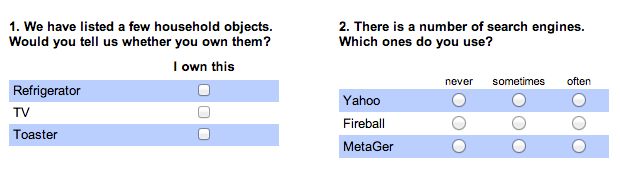### Widths

Browsers may not be able to comply with the specified column widths. This is the case when the cell content is wider than the specified column width. In this case you may solve the problem by the following steps:

• Does the text contain a word that is too long? You can break the word by using `<br>`.
```Lorem ipsum dolor sit amet, consetetur-<br>sadipscingelitrsed
diam nonumy eirmod temporinvi-<br>dutlabore et dolore magna
aliquyam erat```
• Was an image not scaled correctly? Before uploading, images need to be scaled to the correct size. Be aware, browsers recognize the size in pixels only. For more information on this, see Pictures in the questionnaire
Hint: When uploading a different picture with the same file name as an old version, browsers may use the old version from their cache. Try to reload the page.
• If the column widths are still not complied with, you can enforce the browser to do so by using CSS. Add the attribute `style` to the `<table>`-tag:
```<table cellspacing="0" cellpadding="0" border="0" style="table-layout:fixed; width: 100%">
<colgroup>   <!-- The following widths are exactly observed -->
<col width="33%">
<col width="33%">
<col width="34%">
</colgroup>
<!-- etc ... -->```